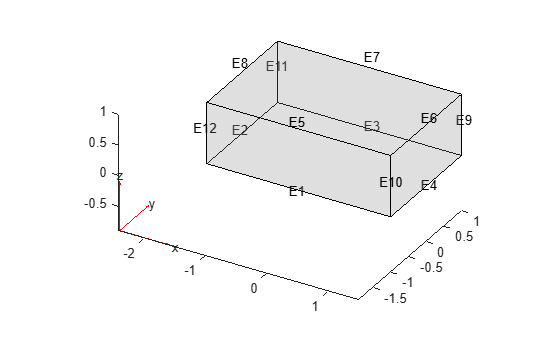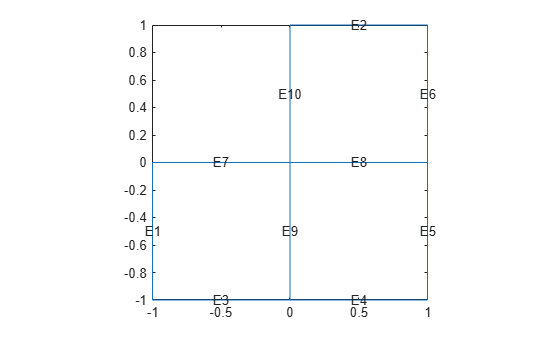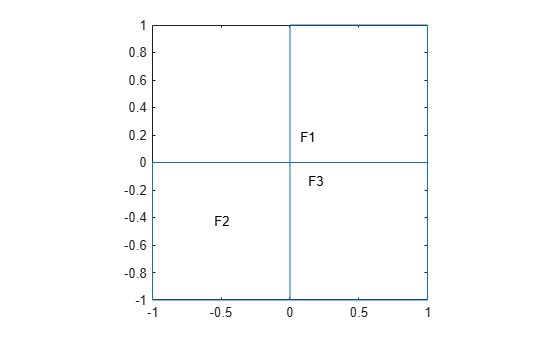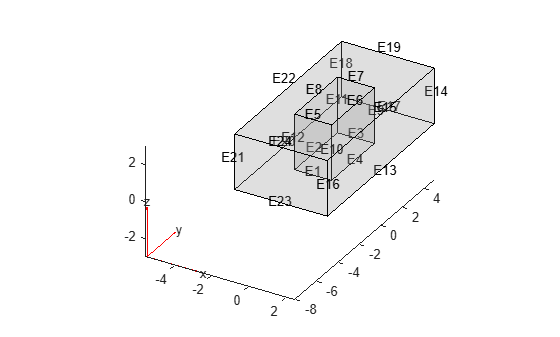# facesAttachedToEdges

Find faces attached to specified edges

## Syntax

``FaceID = facesAttachedToEdges(g,RegionID)``
``FaceID = facesAttachedToEdges(g,RegionID,FilterType)``

## Description

example

````FaceID = facesAttachedToEdges(g,RegionID)` finds faces attached to the edges with ID numbers listed in `RegionID`.```

example

````FaceID = facesAttachedToEdges(g,RegionID,FilterType)` returns internal, external, or all faces attached to the edges with ID numbers listed in `RegionID`. This syntax is valid for 3-D geometries only.```

## Examples

collapse all

Find faces attached to particular edges of a block.

Create a block geometry.

`gm = multicuboid(3,2,1)`
```gm = DiscreteGeometry with properties: NumCells: 1 NumFaces: 6 NumEdges: 12 NumVertices: 8 Vertices: [8x3 double] ```

Plot the geometry with the edge labels.

`pdegplot(gm,'EdgeLabels','on','FaceAlpha',0.2)`Find faces attached to edges 1, 2, and 5.

`faceIDs = facesAttachedToEdges(gm,[1 2 5])`
```faceIDs = 1×4 1 2 5 6 ```

Plot the geometry with the face labels.

```figure pdegplot(gm,'FaceLabels','on','FaceAlpha',0.2)```Find faces attached to particular edges of the L-shaped membrane.

Create a model and include this geometry. The geometry of the L-shaped membrane is described in the file `lshapeg`.

```model = createpde(); gm = geometryFromEdges(model,@lshapeg)```
```gm = AnalyticGeometry with properties: NumCells: 0 NumFaces: 3 NumEdges: 10 NumVertices: 8 Vertices: [8x2 double] ```

Plot the geometry with the edge labels.

`pdegplot(gm,'EdgeLabels','on')`Find faces attached to edges 7 and 10.

`faceIDs = facesAttachedToEdges(gm,[7 10])`
```faceIDs = 1×2 1 2 ```

Plot the geometry with the face labels.

```figure pdegplot(gm,'FaceLabels','on')```Find internal and external faces attached to the edges of the inner cuboid in a geometry consisting of two nested cuboids.

Create a geometry that consists of two nested cuboids of the same height.

`gm = multicuboid([2 5],[4 10],3)`
```gm = DiscreteGeometry with properties: NumCells: 2 NumFaces: 12 NumEdges: 24 NumVertices: 16 Vertices: [16x3 double] ```

Plot the geometry with the edge labels.

`pdegplot(gm,'EdgeLabels','on','FaceAlpha',0.2)`Find all faces attached to the top edges of the inner cuboid.

`facesAttachedToEdges(gm,[5:8])`
```ans = 1×6 2 3 4 5 6 12 ```

Find only the internal faces attached to the top edges of the inner cuboid. Internal faces are faces shared between multiple cells.

`facesAttachedToEdges(gm,[5:8],'internal')`
```ans = 1×4 3 4 5 6 ```

Find only the external faces attached to the top edges of the inner cuboid.

`facesAttachedToEdges(gm,[5:8],'external')`
```ans = 1×2 2 12 ```

Plot the geometry with the face labels.

```figure pdegplot(gm,'FaceLabels','on','FaceAlpha',0.2)```## Input Arguments

collapse all

Geometry, specified as a `DiscreteGeometry` or `AnalyticGeometry` object.

Edge ID, specified as a positive number or a vector of positive numbers. Each number represents an edge ID.

Type of faces to return, specified as `'internal'`, `'external'`, or `'all'`. Depending on this argument, `facesAttachedToEdges` returns these types of faces for a 3-D geometry:

• `'internal'` — Internal faces, that is, faces shared between multiple cells.

• `'external'` — External faces, that is, faces not shared between multiple cells.

• `'all'` — All faces attached to the specified cells.

## Output Arguments

collapse all

IDs of faces attached to the specified edges, returned as a positive number or a vector of positive numbers.